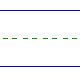Name: ______________________________________________ Date: _____________

Instructions: Find the sum.

 4 + 9 =2 + 2 =7 + 1 =3 + 4 =2 + 6 =8 + 5 =5 + 8 =3 + 1 =1 + 4 =9 + 2 =4 + 0 =4 + 8 =9 + 4 =8 + 1 =5 + 0 =2 + 0 =8 + 3 =7 + 5 =